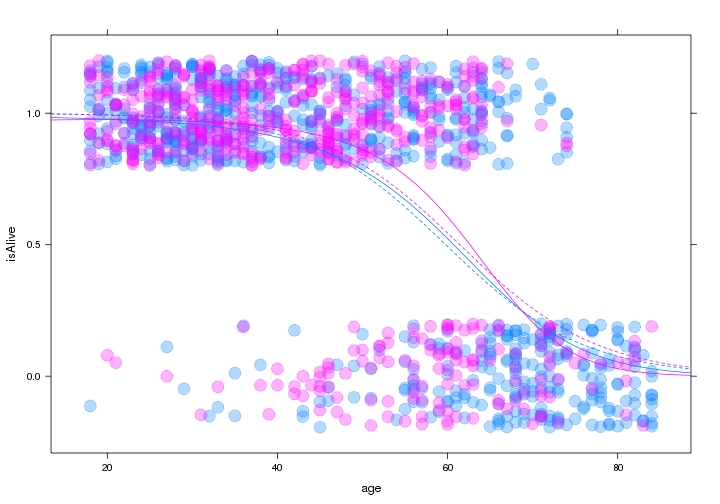# MTH247: Introduction to Regression Analysis

### MLR: Logistic Regression – Fall 2012 – Prof. Baumer

require(mosaic)
require(lmtest)
palette = trellis.par.get()$superpose.symbol$col


Often, we want to model a response variable that is binary, meaning that it can take on only two possible outcomes. These outcomes could be labelled “Yes” or “No”, or “True” of “False”, but for all intents and purposes, they can be coded as either 0 or 1. We have seen these types of variables before (as indicator variables), but we always used them as explanatory variables. Creating a model for such a variable as the response requires a more sophisticated technique than ordinary least squares regression. It requires the use of a logistic model.

The data in the Whickham data set (built into mosaic) contains observations about women, and whether they were alive 20 years after their initial observation. Our goal is to determine how being a $$smoker$$ affects the probability of being alive, after controlling for $$age$$.

data(Whickham)
head(Whickham)

##   outcome smoker age
## 1   Alive    Yes  23
## 2   Alive    Yes  18
## 3    Dead    Yes  71
## 4   Alive     No  67
## 5   Alive     No  64
## 6   Alive    Yes  38


First, let's plot the data. Already we meet challenges.

plotPoints(outcome ~ age, groups = smoker, data = Whickham, alpha = 0.3, pch = 19,
cex = 2)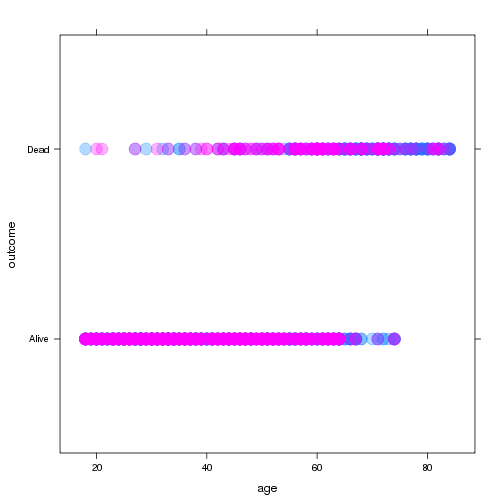It is very difficult to tell what, if anything, is happening here. First, let's add the numeric equivalent for the $$outcome$$ variable.

Whickham = transform(Whickham, isAlive = 2 - as.numeric(outcome))


We can do a little bit better by jittering the points in the $$y$$-direction.

myplot = plotPoints(jitter(isAlive) ~ age, groups = smoker, data = Whickham,
alpha = 0.3, pch = 19, cex = 2, ylab = "isAlive")
print(myplot)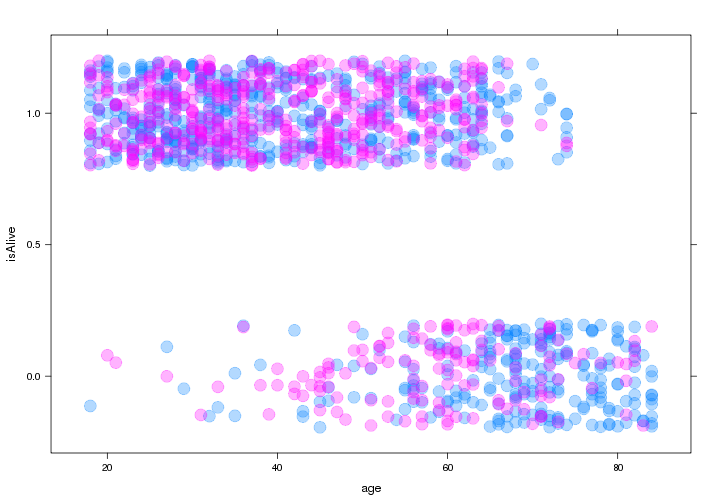A simple approach to building a model here would just be to assign the average to everyone.

print(myplot)
ladd(panel.abline(h = mean(Whickham$isAlive), col = palette))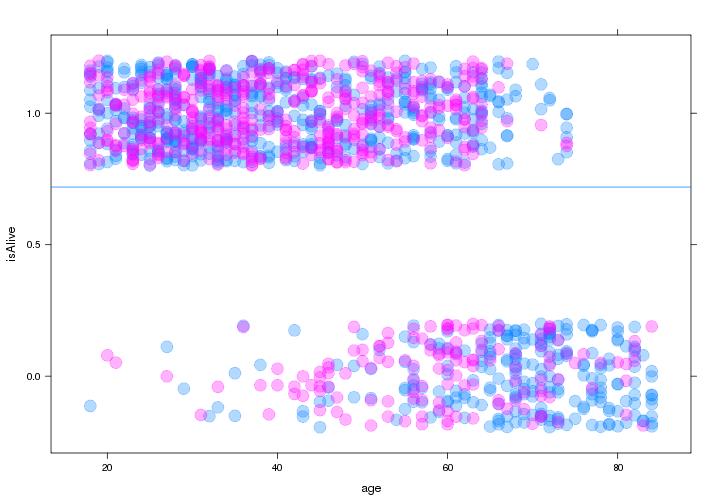Certainly we can improve on this with a linear model, but is it appropriate here? Let's try one. fm = lm(isAlive ~ age + smoker, data = Whickham) summary(fm)  ## ## Call: ## lm(formula = isAlive ~ age + smoker, data = Whickham) ## ## Residuals: ## Min 1Q Median 3Q Max ## -1.1818 -0.1922 0.0178 0.2601 0.7229 ## ## Coefficients: ## Estimate Std. Error t value Pr(>|t|) ## (Intercept) 1.472554 0.030102 48.92 <2e-16 *** ## age -0.016155 0.000558 -28.95 <2e-16 *** ## smokerYes 0.010474 0.019577 0.54 0.59 ## --- ## Signif. codes: 0 '***' 0.001 '**' 0.01 '*' 0.05 '.' 0.1 ' ' 1 ## ## Residual standard error: 0.35 on 1311 degrees of freedom ## Multiple R-squared: 0.394, Adjusted R-squared: 0.393 ## F-statistic: 427 on 2 and 1311 DF, p-value: <2e-16  • Interpret the coefficients of this model. Do they makes sense? • What sort of predictions does this model make? fit.alive = makeFun(fm) print(myplot) plotFun(fit.alive(age = x, smoker = "Yes") ~ x, add = TRUE) plotFun(fit.alive(age = x, smoker = "No") ~ x, col = palette, add = TRUE)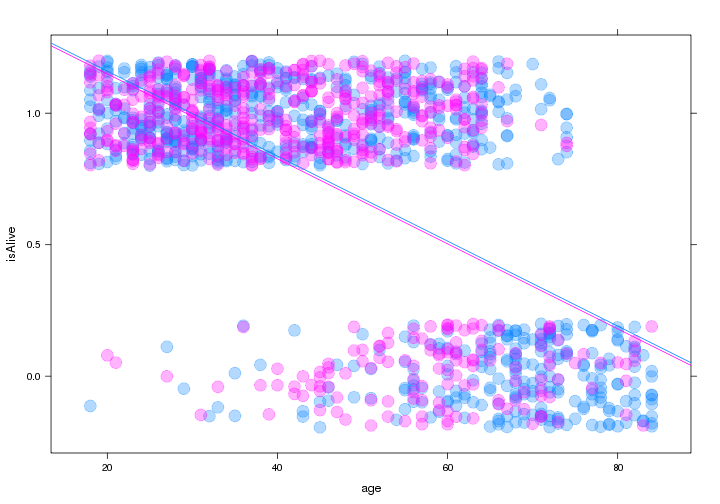#### Fitting a Logistic model Let $$\pi$$ represent the response variable. Then suppose that instead of modeling $\pi = \beta_0 + \beta_1 X$ we modeled $\log \left( \frac{\pi}{1-\pi} \right) = logit(\pi) = \beta_0 + \beta_1 X$ This transformation is called the logit function. What are the properties of this function? Note that this implies that $\pi = \frac{e^{\beta_0 + \beta_1 X}}{1 + e^{\beta_0 + \beta_1 X}} \in (0,1)$ The logit function constrains the fitted values to line within $$(0,1)$$, which helps to give a natural interpretation as the probability of the response actually being 1. Fitting a logistic curve is mathematically more complicated than fitting a least squares regression, but the syntax in R is similar, as is the output. The procedure for fitting is called maximum likelihood estimation, and the usual machinery for the sum of squares breaks down. Consequently, there is no notion of $$R^2$$, etc. # Note that you can also just say 'family=binomial' since logit is the # default option logm = glm(isAlive ~ age + smoker, data = Whickham, family = binomial(logit)) summary(logm)  ## ## Call: ## glm(formula = isAlive ~ age + smoker, family = binomial(logit), ## data = Whickham) ## ## Deviance Residuals: ## Min 1Q Median 3Q Max ## -3.279 -0.438 0.223 0.546 1.958 ## ## Coefficients: ## Estimate Std. Error z value Pr(>|z|) ## (Intercept) 7.59922 0.44123 17.22 <2e-16 *** ## age -0.12368 0.00718 -17.23 <2e-16 *** ## smokerYes -0.20470 0.16842 -1.22 0.22 ## --- ## Signif. codes: 0 '***' 0.001 '**' 0.01 '*' 0.05 '.' 0.1 ' ' 1 ## ## (Dispersion parameter for binomial family taken to be 1) ## ## Null deviance: 1560.32 on 1313 degrees of freedom ## Residual deviance: 945.02 on 1311 degrees of freedom ## AIC: 951 ## ## Number of Fisher Scoring iterations: 6  How can we interpret the coefficients of this model? • Think about the proper interpretation for the coefficients of this model in the context of the problem. The procedure for adding the logistic curve to the plot is the same as it was before. print(myplot) fit.outcome = makeFun(logm) plotFun(fit.outcome(age = x, smoker = "Yes") ~ x, add = TRUE) plotFun(fit.outcome(age = x, smoker = "No") ~ x, col = palette, add = TRUE)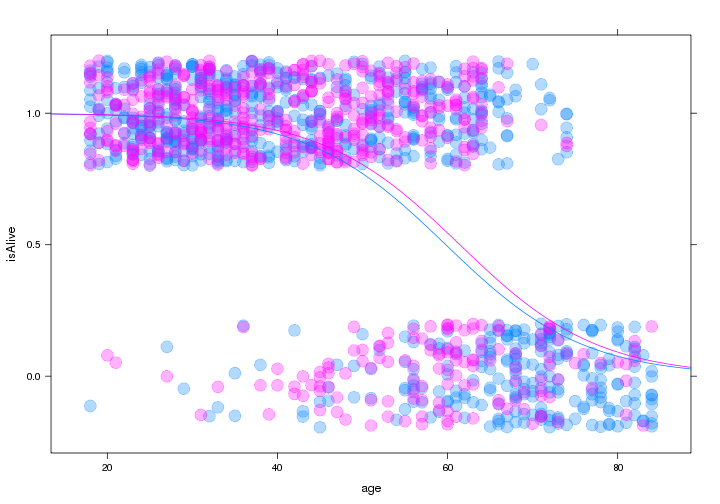• How does the $$smoker$$ term affect the model? ##### Binning Another way to make sense of a binary response variable is to bin the explanatory variable and then compute the average proportion of the response within each bin. Whickham = transform(Whickham, ageGroup = cut(Whickham$age, breaks = 10))
favstats(~isAlive | ageGroup, data = Whickham)

##             min Q1 median Q3 max   mean     sd   n missing
## (17.9,24.5]   0  1      1  1   1 0.9764 0.1525 127       0
## (24.5,31.2]   0  1      1  1   1 0.9791 0.1436 191       0
## (31.2,37.8]   0  1      1  1   1 0.9568 0.2040 162       0
## (37.8,44.4]   0  1      1  1   1 0.9097 0.2876 144       0
## (44.4,51]     0  1      1  1   1 0.8013 0.4003 151       0
## (51,57.6]     0  0      1  1   1 0.7109 0.4551 128       0
## (57.6,64.2]   0  0      1  1   1 0.6071 0.4898 168       0
## (64.2,70.8]   0  0      0  0   1 0.2283 0.4220  92       0
## (70.8,77.5]   0  0      0  0   1 0.1383 0.3471  94       0
## (77.5,84.1]   0  0      0  0   0 0.0000 0.0000  57       0


Although this is not the preferred method for performing logistic regression, it can be illustrative to see how the logistic curve fits through this series of points.

# print(myplot)
binned.y = mean(~isAlive | ageGroup, data = Whickham)
binned.x = mean(~age | ageGroup, data = Whickham)
plotPoints(binned.y ~ binned.x, cex = 2, pch = 19, col = "orange")
plotFun(fit.outcome(age = x, smoker = "Yes") ~ x, add = TRUE)
plotFun(fit.outcome(age = x, smoker = "No") ~ x, col = palette, add = TRUE)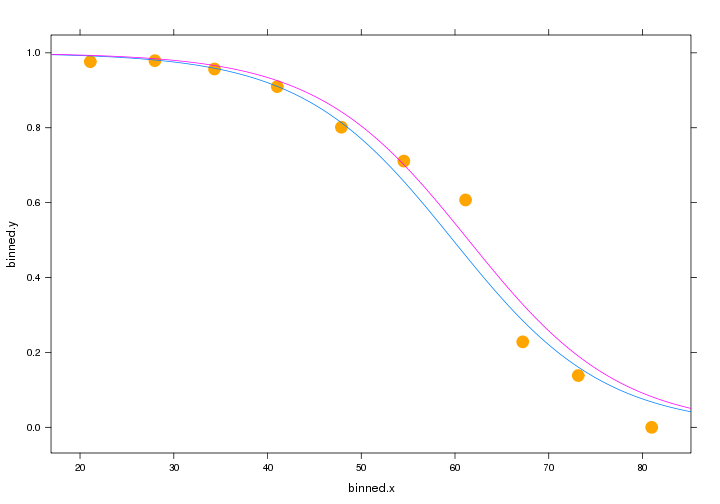##### The Link Values

Consider now the difference between the fitted values and the link values. Although the fitted values do not follow a linear pattern with respect to the explanatory variable, the link values do. To see this, let's plot them explicitly against the logit of the binned values.

plotPoints(logit(binned.y) ~ binned.x, pch = 19, cex = 2, col = "orange")
Whickhamlogm.link = predict(logm, type = "link") plotPoints(logm.link ~ age, data = subset(Whickham, smoker == "Yes"), add = TRUE) plotPoints(logm.link ~ age, data = subset(Whickham, smoker == "No"), add = TRUE, col = palette)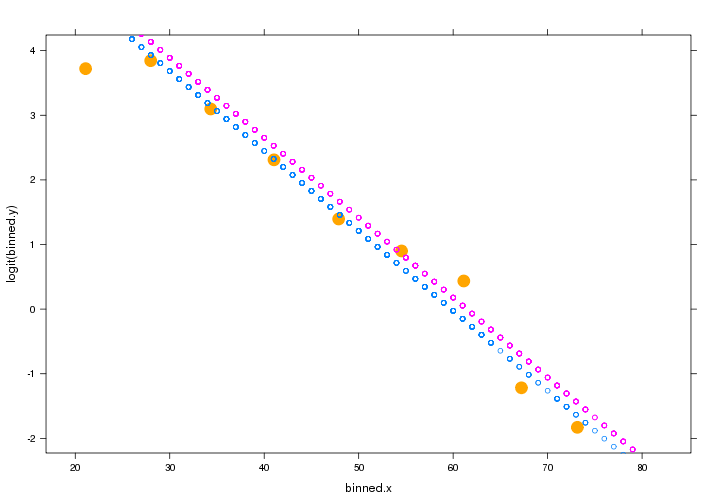Note how it is considerably easier for us to assess the quality of the fit visually using the link values, as opposed to the binned probabilities. • Why can't we take the logit of the actual responses? • Fit a logistic model for $$Acceptance$$ as a function of $$GPA$$, with $$Gender$$ as a covariate using the MedGPA data set. MedGPA = read.csv("http://www.math.smith.edu/~bbaumer/mth247/MedGPA.csv")  #### Odds Ratios and Interpretation of Coefficients The interpretation of the coefficients in a linear regression model are clear based on an understanding of the geometry of the regression model. We use the terms intercept and slope because a simple linear regression model is a line. In a simple logistic model, the line is transformed by the logit function. How do the coefficients affect the shape of the curve in a logistic model? The following manipulate function will allow you to experiment with changes to the intercept and slope coefficients in the simple logistic model for $$isAlive$$ as a function of $$age$$. log.whickham = function(intercept.offset = 0, slope.multiple = 1, ...) { # data(Whickham) Whickham = transform(Whickham, isAlive = 2 - as.numeric(outcome)) logm = glm(isAlive ~ age, data = Whickham, family = binomial(logit)) fit.outcome = makeFun(logm) xyplot(jitter(isAlive) ~ age, groups = smoker, data = Whickham, ylab = "isAlive", panel = function(x, y, ...) { panel.xyplot(x, y, alpha = 0.3, pch = 19, cex = 2, ...) panel.curve(fit.outcome(x), col = "darkgray", lty = 3) panel.curve(fit.outcome(x * slope.multiple + intercept.offset)) }) } require(manipulate) manipulate(log.whickham(intercept.offset, slope.multiple), intercept.offset = slider(-20, 20, step = 1, initial = 0), slope.multiple = slider(0, 5, step = 0.25, initial = 1))  • How do changes in the intercept term affect the shape of the logistic curve? • How do changes in the slope term affect the shape of the logistic curve? We saw earlier that the link values are linear with respect to the explanatory variable. The link values are the $$\log$$ of the odds. Note that if an event occurs with proability $$\pi$$, then $odds = \frac{\pi}{1-\pi}, \qquad \pi = \frac{odds}{1+odds}.$ Note that while $$\pi \in [0,1]$$, $$odds \in (0,\infty)$$. Thus, we can interpret $$\hat{\beta_1}$$ as the typical change in $$\log{(odds)}$$ for each one unit increase in the explanatory variable. More naturally, the odds of success are multiplied by $$e^{\hat{\beta_1}}$$ for each one unit increase in the explanatory variable, since this is the odds ratio. \begin{aligned} odds_X &= \frac{\hat{\pi}_X}{1 - \hat{\pi}_X} = e^{\hat{\beta}_0 + \hat{\beta}_1 X} \\ odds_{X+1} &= \frac{\hat{\pi}_{X+1}}{1 - \hat{\pi}_{X+1}} = e^{\hat{\beta}_0 + \hat{\beta}_1 (X + 1)} \\ \frac{odds_{X+1}}{odds_X} &= \frac{e^{\hat{\beta}_0 + \hat{\beta}_1 (X + 1)}}{e^{\hat{\beta}_0 + \hat{\beta}_1 X}} = e^{\hat{\beta}_1} \end{aligned} Furthermore, since the logits are linear with respect to the explanatory variable, and these changes are constant. Finding confidence intervals for the odds ratio is easy. exp(confint(logm))  ## Waiting for profiling to be done...  ## 2.5 % 97.5 % ## (Intercept) 870.1646 4916.0148 ## age 0.8708 0.8957 ## smokerYes 0.5845 1.1319  • Find confidence intervals for the odds ratios of the terms in the MedGPA model you built earlier. #### Assessing a Logistic Fit Three of the conditions we require for linear regression have analogs for logistic models: • Linearity of the logit (or $$\log{(odds)}$$) • Independence • Random However, the requirements for Constant Variance and Normality are no longer applicable. In the first case, the variability in the response now inherently depends on the value, so we know we won't have constant variance. In the second case, there is no reason to think that the residuals will be normally distributed, since the “residuals” are can only be computed in relation to 0 or 1. So in both cases the properties of a binary response variable break down the assumptions we made previously. Moreover, since we don't have any sums of squares, we can't use $$R^2$$, ANOVA, or $$F$$-tests. Instead, since we fit the model using maximum likelihood estimation, we compute the likelihood of our model. $L(y_i) = \begin{cases} \hat{\pi} & \text{if } y_i=1 \\ 1-\hat{\pi} & \text{if } y_i=0 \end{cases},\qquad L(model) = \prod_{i=1}^n L(y_i)$ Because these numbers are usually very small (why?), it is more convenient to speak of the log-likelihood $$\log(L)$$, which is always negative. A larger $$\log(L)$$ is closer to zero and therefore a better fit. The log-likelihood is easy to retrieve logLik(logm)  ## 'log Lik.' -472.5 (df=3)  but is nearly as easy to calculate directly. pi = logmfitted.values
likelihood = ifelse(Whickham\$isAlive == 1, pi, 1 - pi)
log(prod(likelihood))

##  -472.5


The closest thing to an analog of the $$F$$-test is the Likelihood Ratio Test (LRT). Here, our goal is to compare the log-likelihoods of two models: the one we build vs. the constant model. This is similar to the way we compared the sum of the squares explained by a linear regression model to the model that consists solely of the grand mean.

The null hypothesis in the LRT is that $$\beta_1 = \beta_2 = \cdots = \beta_k = 0$$. The alternative hypothesis is that at least one of these coefficients is non-zero. The test statistic is:
$G = -2\log(constant model) - (-2 \log(model)).$
These two quantities are known as deviances. It can be shown that $$G$$ follows a $$\chi^2$$ distribution with $$k$$ degrees of freedom. This allows us to compute a $$p$$-value for the model.

lrtest(logm)

## Likelihood ratio test
##
## Model 1: isAlive ~ age + smoker
## Model 2: isAlive ~ 1
##   #Df LogLik Df Chisq Pr(>Chisq)
## 1   3   -473
## 2   1   -780 -2   615     <2e-16 ***
## ---
## Signif. codes:  0 '***' 0.001 '**' 0.01 '*' 0.05 '.' 0.1 ' ' 1


In this sense the LRT has obvious similarities to the ANOVA table and $$F$$-test. In the same way that we previously performed a nested $$F$$-test to assess the usefulness of a group of predictors, we can perform a nested LRT.

Adding interaction or quadratic terms works in much the same way as it did with linear regression.

linteract = glm(isAlive ~ age + smoker + age * smoker, data = Whickham, family = binomial)
summary(linteract)

##
## Call:
## glm(formula = isAlive ~ age + smoker + age * smoker, family = binomial,
##     data = Whickham)
##
## Deviance Residuals:
##    Min      1Q  Median      3Q     Max
## -3.398  -0.426   0.216   0.560   1.928
##
## Coefficients:
##               Estimate Std. Error z value Pr(>|z|)
## (Intercept)    8.16923    0.60660   13.47   <2e-16 ***
## age           -0.13323    0.00995  -13.39   <2e-16 ***
## smokerYes     -1.45784    0.83723   -1.74    0.082 .
## age:smokerYes  0.02223    0.01449    1.53    0.125
## ---
## Signif. codes:  0 '***' 0.001 '**' 0.01 '*' 0.05 '.' 0.1 ' ' 1
##
## (Dispersion parameter for binomial family taken to be 1)
##
##     Null deviance: 1560.32  on 1313  degrees of freedom
## Residual deviance:  942.68  on 1310  degrees of freedom
## AIC: 950.7
##
## Number of Fisher Scoring iterations: 6

• Interpret the coefficients of this model, and find confidence intervals for them.

Suppose now that we suspect that there are diminishing returns to the extent to which being alive is associated with a person's age. We can easily add quadratic terms.

lquad = glm(isAlive ~ age + smoker + age * smoker + I(age^2) + I(age^2):smoker,
data = Whickham, family = binomial)
summary(lquad)

##
## Call:
## glm(formula = isAlive ~ age + smoker + age * smoker + I(age^2) +
##     I(age^2):smoker, family = binomial, data = Whickham)
##
## Deviance Residuals:
##    Min      1Q  Median      3Q     Max
## -2.752  -0.281   0.268   0.517   2.137
##
## Coefficients:
##                     Estimate Std. Error z value Pr(>|z|)
## (Intercept)         2.987992   1.418159    2.11  0.03512 *
## age                 0.073704   0.057107    1.29  0.19683
## smokerYes           1.503917   2.131156    0.71  0.48039
## I(age^2)           -0.001939   0.000554   -3.50  0.00047 ***
## age:smokerYes      -0.091566   0.086646   -1.06  0.29061
## smokerYes:I(age^2)  0.001014   0.000856    1.19  0.23588
## ---
## Signif. codes:  0 '***' 0.001 '**' 0.01 '*' 0.05 '.' 0.1 ' ' 1
##
## (Dispersion parameter for binomial family taken to be 1)
##
##     Null deviance: 1560.32  on 1313  degrees of freedom
## Residual deviance:  928.94  on 1308  degrees of freedom
## AIC: 940.9
##
## Number of Fisher Scoring iterations: 6

• Interpret the coefficients of this model, and find confidence intervals for them.

How can we assess whether these terms are warranted? Just like the nested $$F$$-test, the nested LRT gives us information about the incremental contribution of a set of terms to our model.

lrtest(logm, linteract, lquad)

## Likelihood ratio test
##
## Model 1: isAlive ~ age + smoker
## Model 2: isAlive ~ age + smoker + age * smoker
## Model 3: isAlive ~ age + smoker + age * smoker + I(age^2) + I(age^2):smoker
##   #Df LogLik Df Chisq Pr(>Chisq)
## 1   3   -473
## 2   4   -471  1  2.34      0.126
## 3   6   -464  2 13.74      0.001 **
## ---
## Signif. codes:  0 '***' 0.001 '**' 0.01 '*' 0.05 '.' 0.1 ' ' 1

• Experiment with different models until you find one that you think fits the data best. Justify your answer.
print(myplot)
fit.qalive = makeFun(lquad)
plotFun(fit.outcome(age = x, smoker = "Yes") ~ x, add = TRUE, lty = 2)
plotFun(fit.outcome(age = x, smoker = "No") ~ x, col = palette, add = TRUE,
lty = 2)
plotFun(fit.qalive(age = x, smoker = "Yes") ~ x, add = TRUE)
plotFun(fit.qalive(age = x, smoker = "No") ~ x, col = palette, add = TRUE)